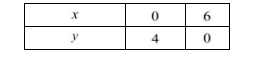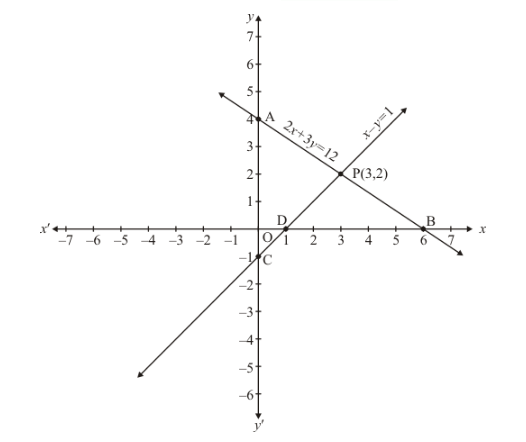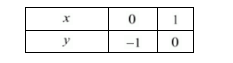# Draw the graphs of the following equations on the same graph paper.

Question:

Draw the graphs of the following equations on the same graph paper.

$2 x+3 y=12$

$x-y=1$

Find the coordinates of the vertices of the triangle formed by the two straight lines and the y-axis.

Solution:

The given equations are

$2 x+3 y=12$$\ldots(i)$

$x-y=1$...(ii)

Putting $x=0$ in equation $(i)$, we get:

$\Rightarrow 2 \times 0+3 y=12$

$\Rightarrow y=4$

$x=0, \quad y=4$

Putting $y=0$ in equation $(i,$, we get:

$\Rightarrow x+3 \times 0=6$

$\Rightarrow x=6$

$x=6, \quad y=0$

Use the following table to draw the graph.Draw the graph by plotting the two points $A(0,2)$ and $B(6,0)$ from table.$x-y=1$ ... (ii)

Putting $x=0$ in equation (ii) we get:

$\Rightarrow 0-y=1$

$\Rightarrow y=-1$

$x=0, \quad y=-1$

Putting $y=0$ in equation $(i i)$, we get:

$\Rightarrow x-0=1$

$\Rightarrow x=1$

$x=1, \quad y=0$

Use the following table to draw the graph.Draw the graph by plotting the two points $C(0,-1), D(1,0)$ from table.

Draw the graph by plotting the two points from table.

The intersection point is P(3, 2)

Three points of the triangle are $A(0,4), C(0,-1)$ and $P(3,2)$.

Hence the value of $x=3$ and $y=2$